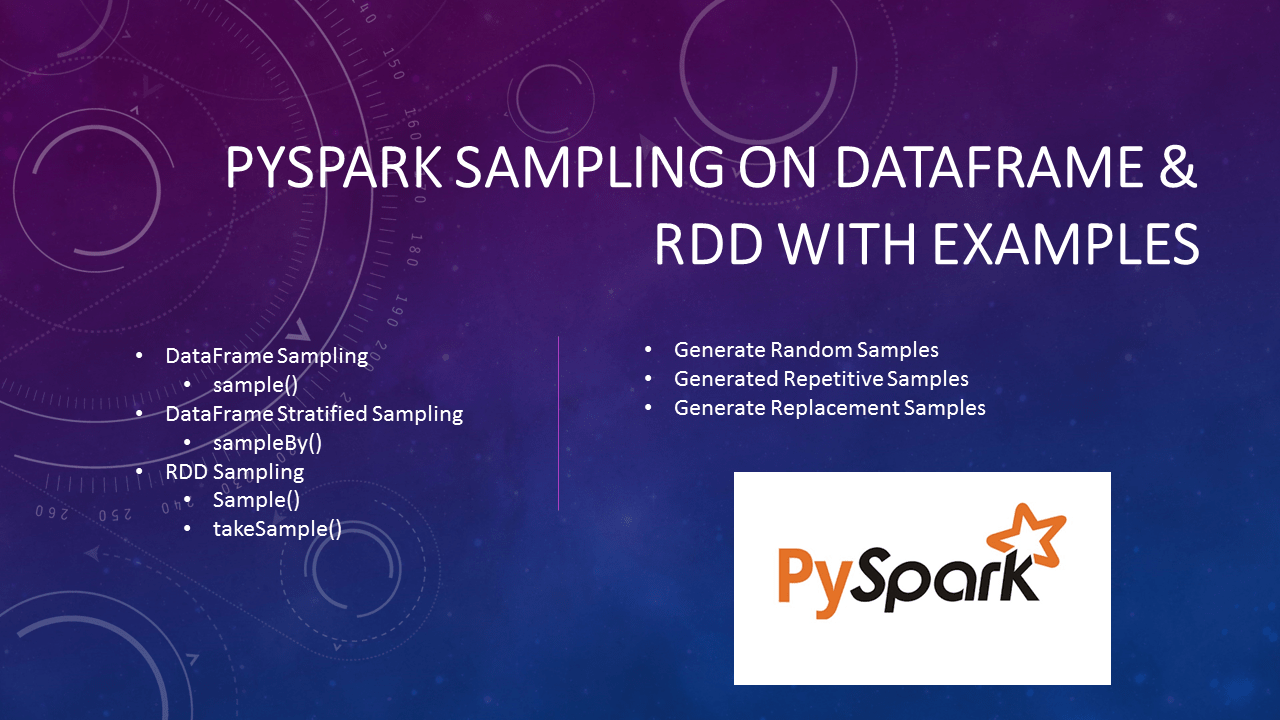PySpark Random Sample with ExamplePySpark provides a pyspark.sql.DataFrame.sample(), pyspark.sql.DataFrame.sampleBy(), RDD.sample(), and RDD.takeSample() methods to get the random sampling subset from the large dataset, In this article I will explain with Python examples .

If you are working as a Data Scientist or Data analyst you often required to analyze a large dataset/file with billions or trillions of records, processing these large datasets takes some time hence during the analysis phase it is recommended to use a random subset sample from the large files.

1. PySpark SQL sample() Usage & Examples

PySpark sampling (pyspark.sql.DataFrame.sample()) is a mechanism to get random sample records from the dataset, this is helpful when you have a larger dataset and wanted to analyze/test a subset of the data for example 10% of the original file.

Below is syntax of the sample() function.

sample(withReplacement, fraction, seed=None)

fraction – Fraction of rows to generate, range [0.0, 1.0]. Note that it doesn’t guarantee to provide the exact number of the fraction of records.

seed – Seed for sampling (default a random seed). Used to reproduce the same random sampling.

withReplacement – Sample with replacement or not (default False).

Let’s see some examples.

1.1 Using fraction to get a random sample in PySpark

By using fraction between 0 to 1, it returns the approximate number of the fraction of the dataset. For example, 0.1 returns 10% of the rows. However, this does not guarantee it returns the exact 10% of the records.

Note: If you run these examples on your system, you may see different results.

from pyspark.sql import SparkSession
spark = SparkSession.builder
.master("local")
.appName("SparkByExamples.com")
.getOrCreate()

df=spark.range(100)
print(df.sample(0.06).collect())
//Output: [Row(id=0), Row(id=2), Row(id=17), Row(id=25), Row(id=26), Row(id=44), Row(id=80)]

My DataFrame has 100 records and I wanted to get 6% sample records which are 6 but the sample() function returned 7 records. This proves the sample function doesn’t return the exact fraction specified.

1.2 Using seed to reproduce the same Samples in PySpark

Every time you run a sample() function it returns a different set of sampling records, however sometimes during the development and testing phase you may need to regenerate the same sample every time as you need to compare the results from your previous run. To get consistent same random sampling uses the same slice value for every run. Change slice value to get different results.

print(df.sample(0.1,123).collect())
//Output: 36,37,41,43,56,66,69,75,83
print(df.sample(0.1,123).collect())
//Output: 36,37,41,43,56,66,69,75,83
print(df.sample(0.1,456).collect())
//Output: 19,21,42,48,49,50,75,80

On above examples, first 2 I have used slice 123 hence the sampling results are same and for last I have used 456 as slice hence it has returned different sampling records

1.3 Sample withReplacement (May contain duplicates)

some times you may need to get a random sample with repeated values. By using the value true, results in repeated values.

print(df.sample(True,0.3,123).collect()) //with Duplicates
//Output: 0,5,9,11,14,14,16,17,21,29,33,41,42,52,52,54,58,65,65,71,76,79,85,96
print(df.sample(0.3,123).collect()) // No duplicates
//Output: 0,4,17,19,24,25,26,36,37,41,43,44,53,56,66,68,69,70,71,75,76,78,83,84,88,94,96,97,98

On first example, values 14, 52 and 65 are repeated values.

1.4 Stratified sampling in PySpark

You can get Stratified sampling in PySpark without replacement by using sampleBy() method. It returns a sampling fraction for each stratum. If a stratum is not specified, it takes zero as the default.

sampleBy() Syntax

sampleBy(col, fractions, seed=None)

col – column name from DataFrame

fractions – It’s Dictionary type takes key and value.

sampleBy() Example

df2=df.select((df.id % 3).alias("key"))
print(df2.sampleBy("key", {0: 0.1, 1: 0.2},0).collect())
//Output: [Row(key=0), Row(key=1), Row(key=1), Row(key=1), Row(key=0), Row(key=1), Row(key=1), Row(key=0), Row(key=1), Row(key=1), Row(key=1)]

2. PySpark RDD Sample

PySpark RDD also provides sample() function to get a random sampling, it also has another signature takeSample() that returns an Array[T].

RDD sample() Syntax & Example

PySpark RDD sample() function returns the random sampling similar to DataFrame and takes a similar types of parameters but in a different order. Since I’ve already covered the explanation of these parameters on DataFrame, I will not be repeating the explanation on RDD, If not already read I recommend reading the DataFrame section above.

sample() of RDD returns a new RDD by selecting random sampling. Below is a syntax.

sample(self, withReplacement, fraction, seed=None)

Below is an example of RDD sample() function

rdd = spark.sparkContext.range(0,100)
print(rdd.sample(False,0.1,0).collect())
//Output: [24, 29, 41, 64, 86]
print(rdd.sample(True,0.3,123).collect())
//Output: [0, 11, 13, 14, 16, 18, 21, 23, 27, 31, 32, 32, 48, 49, 49, 53, 54, 72, 74, 77, 77, 83, 88, 91, 93, 98, 99]

RDD takeSample() Syntax & Example

RDD takeSample() is an action hence you need to careful when you use this function as it returns the selected sample records to driver memory. Returning too much data results in an out-of-memory error similar to collect().

Syntax of RDD takeSample() .

takeSample(self, withReplacement, num, seed=None)

Example of RDD takeSample()

print(rdd.takeSample(False,10,0))
//Output: [58, 1, 96, 74, 29, 24, 32, 37, 94, 91]
print(rdd.takeSample(True,30,123))
//Output: [43, 65, 39, 18, 84, 86, 25, 13, 40, 21, 79, 63, 7, 32, 26, 71, 23, 61, 83, 60, 22, 35, 84, 22, 0, 88, 16, 40, 65, 84]

Conclusion

In summary, PySpark sampling can be done on RDD and DataFrame. In order to do sampling, you need to know how much data you wanted to retrieve by specifying fractions.

Use seed to regenerate the same sampling multiple times. and

Use withReplacement if you are okay to repeat the random records.

Thanks for reading. If you recognize my effort or like articles here please do comment or provide any suggestions for improvements in the comments sections!

Reference